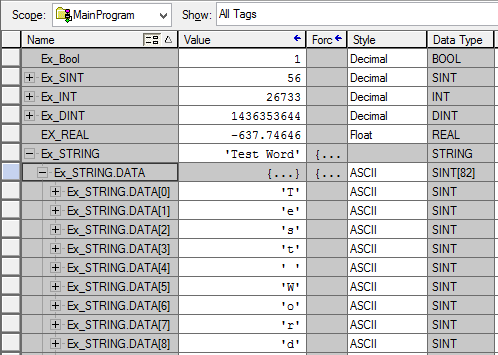### AB PLC Data Types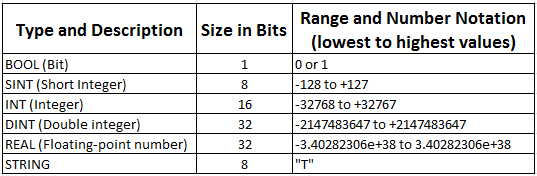### BOOL (Bit)

A Boolean variable, one whose value is either true or false.

Example:- 0 or 1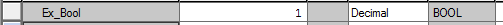### SINT (Short Integer)

Short Integer uses only 8 bits of memory and therefore is a value of 0 to 255 or if signed from -128 to +127.

Example:-  56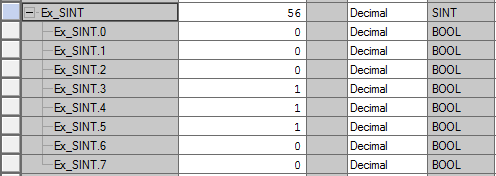### INT (Integer)

Integer uses only 16 bits of memory and therefore is a value of 0 to 65535 or if signed from -32768 to +32767.

Example:- 26733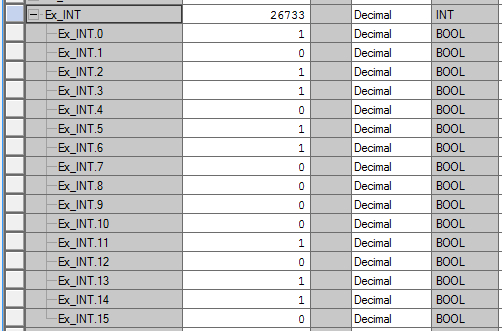### DINT (Double integer)

Double Integer uses only 32 bits of memory and therefore is a value of 0 to 4294967295 or if signed from -2147483647 to +2147483647.

Example:- 1436353644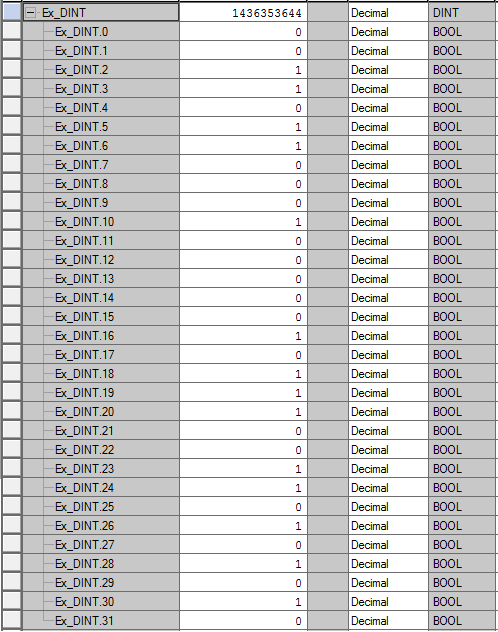### REAL (Floating-point number)

Real uses only 32 bits of memory. Value from -3.40282306e+38  to 3.40282306e+38

Example:-   -637.74646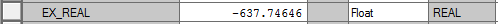### STRING

String uses only 8 bits of memory for single character. If you use 4 character total uses only 32 bits of memory

Example:-   "Test Word
T          - 8 bits
e           - 8 bits
s           - 8 bits
t            - 8 bits
Space   - 8 bits
W         - 8 bits
o           - 8 bits
r            - 8 bits
d           - 8 bits

Total     - 72 bits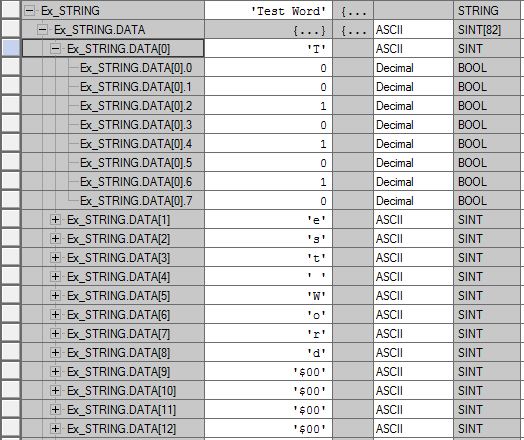#### Example from Rslogix 5000